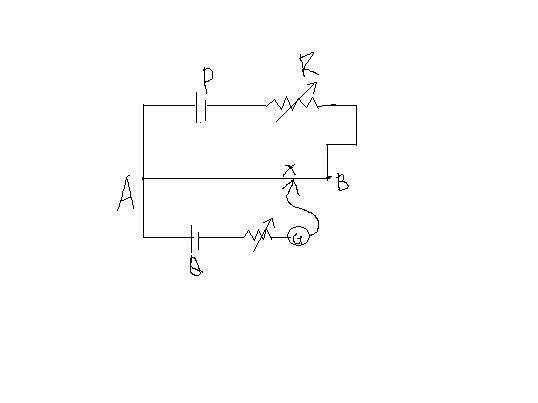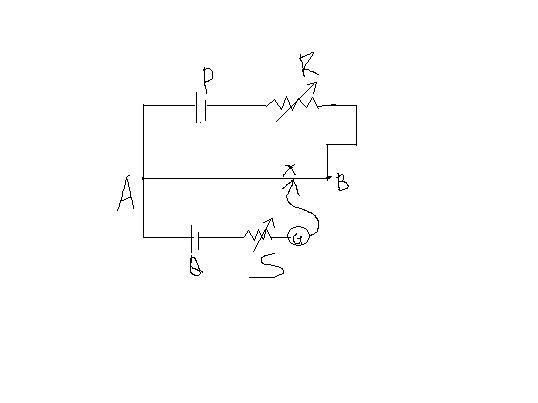# Potentiometer

Q In the potentiometer circuit shown,the balance point is at X.State with reason where the balance point be shifted when: 1)resistance R is increased keeping all other parameters unchanged 2)resistance S is increased keeping R constant 3)cell P is replaced by another cell whose emf is lower than that of cell Q•parthiban ·

1.)When resistance R is increased the current through the potentiometer wire will decrease,due to which,the potential gradient will also decrease.thus,the balance point will shift towards B.
2.)At balance point,current through Q=0.thus no change in balance point.
3.)The potentiometer wouldn't work as the emf of Q will be higher than the overall p.d across the whole wire AB.
Am i right?

•Honey Arora ·

•voldy ·

what's S?

Honey Arora ·

edited..........•Anonymous ·

1) R is increased so I decreases and V decreases so potential gradient(V/l) decreases and hence balance point shifted towards B

2) S is increased so no change

3) emf lower than Q then potentiometer wont work

•